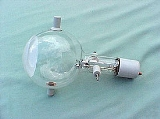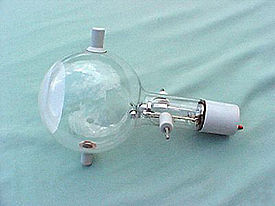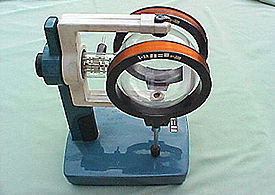xTeltron TubeEncyclopediaA teltron tube is a type of cathode ray tube
Cathode ray tube
The cathode ray tube is a vacuum tube containing an electron gun and a fluorescent screen used to view images. It has a means to accelerate and deflect the electron beam onto the fluorescent screen to create the images. The image may represent electrical waveforms , pictures , radar targets and...

used to demonstrate the properties of electrons. It usually contains two electron gun
Electron gun
An electron gun is an electrical component that produces an electron beam that has a precise kinetic energy and is most often used in television sets and computer displays which use cathode ray tube technology, as well as in other instruments, such as electron microscopes and particle...

s, which can project two thin electron beams at right angles. The beams can be bent by applying voltages to various electrodes in the tube. The electron beams are visible as fine bluish lines. This is accomplished by filling the tube with low pressure helium
Helium
Helium is the chemical element with atomic number 2 and an atomic weight of 4.002602, which is represented by the symbol He. It is a colorless, odorless, tasteless, non-toxic, inert, monatomic gas that heads the noble gas group in the periodic table...

gas. A few of the electrons in the beam collide with the helium atoms, causing them to fluoresce and emit light.

They are usually used to teach electromagnetic effects because they show how an electron beam is affected by electric field
Electric field
In physics, an electric field surrounds electrically charged particles and time-varying magnetic fields. The electric field depicts the force exerted on other electrically charged objects by the electrically charged particle the field is surrounding...

s and by magnetic field
Magnetic field
A magnetic field is a mathematical description of the magnetic influence of electric currents and magnetic materials. The magnetic field at any given point is specified by both a direction and a magnitude ; as such it is a vector field.Technically, a magnetic field is a pseudo vector;...

s.

## Motions in fields

Charged particles in a uniform electric field follow a parabolic trajectory
Parabolic trajectory
In astrodynamics or celestial mechanics a parabolic trajectory is a Kepler orbit with the eccentricity equal to 1. When moving away from the source it is called an escape orbit, otherwise a capture orbit...

, since the electric field
Electric field
In physics, an electric field surrounds electrically charged particles and time-varying magnetic fields. The electric field depicts the force exerted on other electrically charged objects by the electrically charged particle the field is surrounding...

term (of the Lorentz force which acts on the particle) is the product of the particle's charge and the magnitude of the electric field, (oriented in the direction of the electric field). In a uniform magnetic field however, charged particles follow a circular trajectory due to the cross product
Cross product
In mathematics, the cross product, vector product, or Gibbs vector product is a binary operation on two vectors in three-dimensional space. It results in a vector which is perpendicular to both of the vectors being multiplied and normal to the plane containing them...

in the magnetic field
Magnetic field
A magnetic field is a mathematical description of the magnetic influence of electric currents and magnetic materials. The magnetic field at any given point is specified by both a direction and a magnitude ; as such it is a vector field.Technically, a magnetic field is a pseudo vector;...

term of the Lorentz force. (That is, the force from the magnetic field acts on the particle in a direction perpendicular to the particle's direction of motion. See: Lorentz force
Lorentz force
In physics, the Lorentz force is the force on a point charge due to electromagnetic fields. It is given by the following equation in terms of the electric and magnetic fields:...

for more details.)

## The Apparatus

The 'teltron' apparatus consists of a Teltron type electron deflection tube, a Teltron stand, EHT power supply (0 - 5000 V DC, variable).